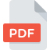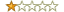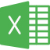# CA Final - SFM

Dividends Theory #pdfon 27 February 2016

690 times
164 KB
Rating:1

Download Other files in Students category

File Content -

C A & C M A Coaching Centre, Nallakunta, Hyderabad. P V Ram, B. Sc., ACA, ACMA – 98481 85073 Score 60+ thro’ SYSTEMATIC & SMART Study Page 1 of 8 CHAPTER 4 : Dividends Dividends are that part of the earnings which are distributed to the members of the company in cash. The balance part of earnings is called retained earnings. Dividend policy: Each organisation has its own policy for dividends and this may change from time to time as well. Essentially there are two type s which a company can adopt: a. Dividend as long term financing policy: Under this policy earnings are treated as a long term source of funds and earnings are utilised to finance the expansion programs. When the firm does not have alternative avenues for investing, then it will distribute the amount as dividends. b. Wealth maximisation policy: Due to market imperfections and uncertainity, shareholders prefer immediate returns than long term gains in the form of capital appreciation. High payouts will result i n higher share prices and vice versa. This may result into losing of fourable alternative investment avenues due to lack of funds. Practical considerations for declaring dividends: Following are the factors that are looked into before declaring dividends. a. Liquidity: The availability of funds with the company and alternative avenues for investing are looked into. b. Repayment of debt obligations, if any : c. Stability and continuity of profits: d. Control : The use of retained earnings to finance new projects preserves the company’s ownership and control. Raising of funds from outside may dilute the ownership e. Legal considerations: Requirements of Companies Act 2013. f. Impact on stock market prices: g. Tax considerations: h. Desire of share holders: i. Stability of dividends: j. Dividend payout ratio: C A & C M A Coaching Centre, Nallakunta, Hyderabad. P V Ram, B. Sc., ACA, ACMA – 98481 85073 Score 60+ thro’ SYSTEMATIC & SMART Study Page 2 of 8 k. Inflation: In periods of high inflation higher dividends are paid to compensate the shareholders for raising prices. Form of dividend: Dividend can be in the form of Cash or in the form of Bonus issues. Bonus issue as such is no dividend at all as this will not improve the value to the shareholders. However, making bonus issue has following advantages: a. The company can conserve cash and utilise it for better investment alternatives. Further, cash outflow can be saved as dividend tax need not be paid. b. In case if a policy of paying fixed dividend is followed by the company, bonus issues will result in higher cash outflows in following years. c. Usually when a bonus issue is made, market share price will come down. But the fall in price will not be in proportion to incre ase in the holding of the members. Thus, the value of holding of the memb ers increases. Stock dividends also have the following limitations: a. Bonus does not add value to shareholders as they do not receive anything and what they receive is already theirs. b. Stock dividends are more costly to administer than cash dividends. Theories on dividend policies : There are several theories on dividend policies. Each theory is made with certain assumptions and lays st ress on certain factors. 1. Traditional theory: Traditional theory lays more emphasis on liberal dividends and says that stock prices react more positively with higher dividends than with lower dividends. As per this: P = m(4D + R) / 3 = m(D + E/3) Where: P = Mkt. Price of share; D = Dividend per share; R = Retained Earnings per share; E= EPS & m = a constant multiplier. Due to the emphasis on dividends, they give four weights for each weight of retained earnings. C A & C M A Coaching Centre, Nallakunta, Hyderabad. P V Ram, B. Sc., ACA, ACMA – 98481 85073 Score 60+ thro’ SYSTEMATIC & SMART Study Page 3 of 8 2. Walter’s Theory: Walter says that share prices are the present values of expected future dividends and retained earnings. His the ory is based on following assumptions: a. The firm is financed only by equity; b. Future investments are made from earnings only; c. Return on investments (r) is constant. d. Life of firm is infinite. As per him: P = [D + r (E - D) / k] / k P = Mkt. Price of share; D = Dividend per share; r = Return on internal retentions; E= EPS & k = Cost of Capital. Walter’s theory emphasises two things: Viz., Dividen d per share (D/P), and cost of capital represented by the capitalisation rate k. The formula itself has two components: D / k – indicating the PV of infinite stream of dividends and [r (E-D) / k] / r – indicating the PV of infinite stream of retained earnings I f r > k, the share price will increase as Div. Payout decrease s. If r = k, then the price will not change with D/P ratio. If r < k, then also share price will increase if Div. Payout increases. Walter’s theory implies that the optimal payout ratio for: A growth co. (i.e r > k) is nil. A normal co. (i.e. r = k ) is irrelevant, and A declining (i.e. r < k) firm is 100% 3. Gordon growth model: This theory is based on capitalisation approach. Assumptions under this theory are: a. The firm is financed only by equity; b. Future investments are made from earnings only; c. Return on investments (r) is constant; d. Cost of capital (k) is constant and greater than growth rate. e. Retention ratio, b once decided remains constant. f. Life of firm is infinite. C A & C M A Coaching Centre, Nallakunta, Hyderabad. P V Ram, B. Sc., ACA, ACMA – 98481 85073 Score 60+ thro’ SYSTEMATIC & SMART Study Page 4 of 8 As per him: P0 = [D o (1 + g)] / (k - g) P 0 = Mkt. Price per share before dividend ; D 0 = current year dividend; g = constant annual growth rate of dividends; k = cost of equity. Another formula of Gordon when growth is included in earnings and dividend, it is : P0 = [E (1 - b)] / (k – b r) P 0 = Mkt. Price per share before dividend; E = EPS, b = % of earnings retained; k = cost of equity; g = growth rate of dividends; and r = Return on internal retentions In case of constant growth: P = D1 / (K – g) In case of zero growth of dividends (i.e. each year, dividend will be fixed amount), the formula is: P = D1 / K P = Mkt. Price; D 1 = Div. In year 1; K = Cost of capital; g = growth rate of dividends. If r > k, the share price will increase as Div. Payout decreases. If r = k, then the price will not change with D/P ratio. If r < k, then also share price will increase if Div. Payout increases. From the above, it can be seen that the assumptions and implications of Gordon model are more or less same as Walter model. 4. Miller and Modigliani (M&M) Model: M & M state that a firm’s dividend policy has no impact on the value of its assets, and so on the market price of share. They state that Under conditions of perfect capital markets, rational investors, absence of tax discrimination between dividend income and capital appreciation, given the firm ’s investment policy, its dividend policy may have no influence on the market price of shares. Firms if do not pay dividends will have higher retained earnings and will either reduce the volume of new stock issues, repurchase more stock from market or simply invest extra cash in marketable securities. In all the above cases, the loss by investors C A & C M A Coaching Centre, Nallakunta, Hyderabad. P V Ram, B. Sc., ACA, ACMA – 98481 85073 Score 60+ thro’ SYSTEMATIC & SMART Study Page 5 of 8 of cash dividends will be made up in the form of capital gains. Assumptions under this hypothesis are: a. Information on capital market is freely available; b. There are no taxes; c. No floatation and transaction costs; d. The firm has a fixed investment policy; e. No risk of uncertainity. f. No investor can sway the market forces; g. Securities are infinitely divisible As per M & M, if dividend is declared, the price of share will be: P0 = (P 1 + D 1) / (1 + K) And if dividend is not declared, it will be: P0 = P 1 / (1 + K) P 0 = Current Mkt. Price of share; P 1 = Mkt. Price at the end of period 1; K= Cost of capital; D 1 = Div. In year 1. Further, if the firm intends to finance all its projects, ΔN = [I – (E – nD 1)] / P1 ΔN = Change in the number of shares; I = Total amount requd. As capital budget; E = PAT; D 1 = Div. In year 1; P 1 = Mkt. Price at the end of period 1; Value of the firm = (No. Of Shares * Mkt. Price + E – Investments) / (1 + K) E = Earnings; K = Cost of Capital. 5. Lintner’s model: Lintner has stated his theory on the basis of his interviews with businessmen. As per his model: D 1 = D 0 + [(EPS * Target payout) – D 0] * A f = D 0 (1 -A f) +EPS * Target payout * A f D 1 = Div. In year 1; D 0 = Div. In year 0; A f = Adj. Factor 6. Radical approach: In this approach, tax aspects on dividends are considered. Presently as dividends and L T capital gains on shares are not taxable, this is not much relevant. However, what it states i s, if tax on dividends is lesser than on capital gains, the share price of the company offering dividends will be priced better and vice versa. 7. Dividend discount model: This is a financial model. Under this C A & C M A Coaching Centre, Nallakunta, Hyderabad. P V Ram, B. Sc., ACA, ACMA – 98481 85073 Score 60+ thro’ SYSTEMATIC & SMART Study Page 6 of 8 model, the price a share will be traded is calculated by the net present value of all expected future divided payment discounted by an appropriate risk-adjusted rate. The required rate of return (capitalization rate) is the rate of return required by investors to compensate them for the risk of owning the security this is similar to calculation of the price of a bond which is the sum of the present value of its future interest payments discounted by the market rate. Future cash flows include dividends and the sale price of the stock when it i s sold. If the stock pays no dividend, then the expected future cash flow is the sale price of the stock. Intrinsic value = Sum of the PVs’ of future cash flows. = PV of dividends + PV of sale value of stock. D1 + D2 + D3 +. . .+ Dn + P = (1+k) 1 (1+k) 2 (1+k) 3 (1+k) n (1+k) n Dn = Dividend of year n ; P = Sale price of stock; n = No. Of yrs. K = Capitalisation rate. Discount model has 3 sub models: a. Zero growth model: Each year dividends are fixed: Then, Intrinsic Value = D / K b. Constant growth (Gordon Model): Then, Intrinsic Value (P) = D 1 / (K – g) P = Mkt. Price; D 1 = Div. In year 1; K = Cost of capital; g = growth rate of dividends. c. Variable growth rate model: Usually the three stages of growth, the initial high rate of growth, a transition to slower growth, and lastly a sustainable steady growth are considered. For each type of stage, appropriate suitable variables are considered and PVs’ are calculated and sum of the three PVs’ is the intrinsi c value of share. 8. H Model: The H model is a two-stage model for growth, the growth rate in the initial growth phase is not constant but declines linearly over time to reach the stable growth rate in steady stage. This model is based on the assumption that the earnings growth rate starts at a high initial rate (g a) and declines linearly over the extraordinary growth period (which is assumed to last 2H periods) to a stable growth rate (g n) (H is the accelerated growth period and declines to normal growth in H further years. So, total time is 2H years). It also assumes C A & C M A Coaching Centre, Nallakunta, Hyderabad. P V Ram, B. Sc., ACA, ACMA – 98481 85073 Score 60+ thro’ SYSTEMATIC & SMART Study Page 7 of 8 that the dividend payout and cost of equity are constant over time and are not affected by the shifting growth rates. Formula used for calculation of price is: � ￿= � ￿￿￿ : ￿� �; �− � � + � ￿￿￿�∗ : � �−� �; �− � � Where, (Stable Growth) (Accelerated Growth) P 0 is Present Value of share; D 0 is Dividend per share; g a is initial growth rate; g n is normal growth rate at the end of 2H years; k is cost of capital. First part of the formula indicates value during stable growth phase and second part of the formula indicates the value during accelerated growth phase. (Alternatively, we may use directly dividend discount model also.) Homemade dividends: In case of Homemade dividends, the holder of shares sells a small portion of his investment to compensate herself for non declaration dividends or reduced declaration of dividends and yet continues to possess the same value of investments as before. Let us assume that Mrs. X holds 80 shares in a company whose share price after it declared a dividend of Rs. 2 per share is Rs. 42 (Cum Div. Price). You would expect the ex-dividend price to become Rs. 40 after the rec ord date. Mrs. X does not like Rs. 2 per share – she had expected Rs. 3 per share and, therefore, she resorts to ‘home made dividends’ Mrs. X’s current wealth is Rs. 42 x 80 = Rs. 3,360 Mrs. X’s post dividend wealth would be Rs. 40 x 80 + Rs. 160 = Rs. 3,360 (i.e. market price + dividend on hand), if she is happy with the Rs. 2 per share dividend. She can derive the same value by selling 2 shares at ex-dividend price of Rs. 40 x 2 = Rs. 80. Her wealth now is Rs. 40 x 78 + Rs. 160 + Rs. 8 0 = Rs. 3,360 If the company, in deference to the wishes of Mrs. X had declared Rs. 3 per share as dividend, her post dividend wealth would be Rs. 39x80 + Rs. 240 = Rs. 3,360 In all cases above, the wealth of the shareholder never changed, in sp ite of the fact that the amount of dividend varies. This supports M M Theory. C A & C M A Coaching Centre, Nallakunta, Hyderabad. P V Ram, B. Sc., ACA, ACMA – 98481 85073 Score 60+ thro’ SYSTEMATIC & SMART Study Page 8 of 8 Questions: 1. Write short note on effect of a Government imposed freeze on dividends on stock prices and the volume of capital investment in the background of Miller-Modigliani (MM) theory on dividend policy. 2. Write short note on factors determining the dividend policy of a company. 3. What are the determinants of Dividend Policy? 4. How tax considerations are relevant in the context of a dividend decision of a company? 5. According to the position taken by Miller and Modigliani, d ividend decision does not influence value. Please state briefly any two reasons, why companies should declare dividend and not ignore it.

#### Trending Downloads##### GST Compliance Reco 2021-22
On : 12 June 2021
Download##### How to calculate the value of a business/enterprise
On : 12 June 2021
Download##### Comparison of sections 194Q with 206C(1H)
On : 12 June 2021
Download##### Excel Voucher
On : 16 June 2021
Download##### Break Even Analysis Chart
On : 14 June 2021
Download##### ICAI leads in capacity building of profession through digital audit tools
On : 12 June 2021
Download

More Options »